# RD Sharma Solutions for Class 6 Maths Chapter 4: Operations on Whole Numbers Exercise 4.2

## RD Sharma Solutions for Class 6 Maths Exercise 4.2 PDF

Subject faculty at BYJU’S creates the chapter wise solutions which can be used as a reference guide to ace the exam. The primary focus is to create accurate solutions which are easy for the students to understand. The detailed and stepwise explanation for each answer of the exercise wise problems are based on the CBSE guidelines. The solutions are prepared by subject experts with the aim of helping students revise the entire syllabus and perform well in the exam. RD Sharma Solutions for Class 6 Maths Chapter 4 Operations on Whole Numbers Exercise 4.2 are provided here.

## RD Sharma Solutions for Class 6 Maths Chapter 4: Operations on Whole Numbers Exercise 4.2 Download PDF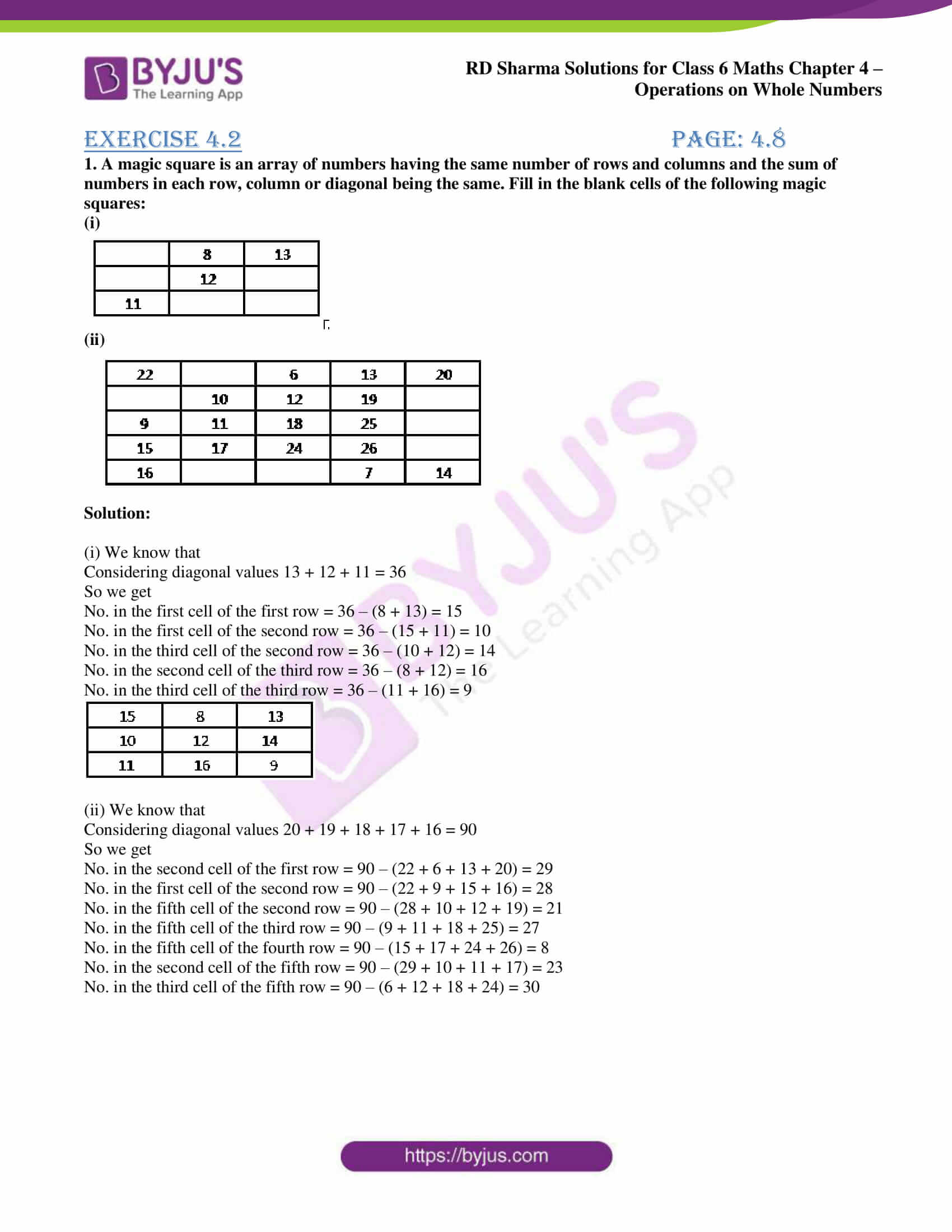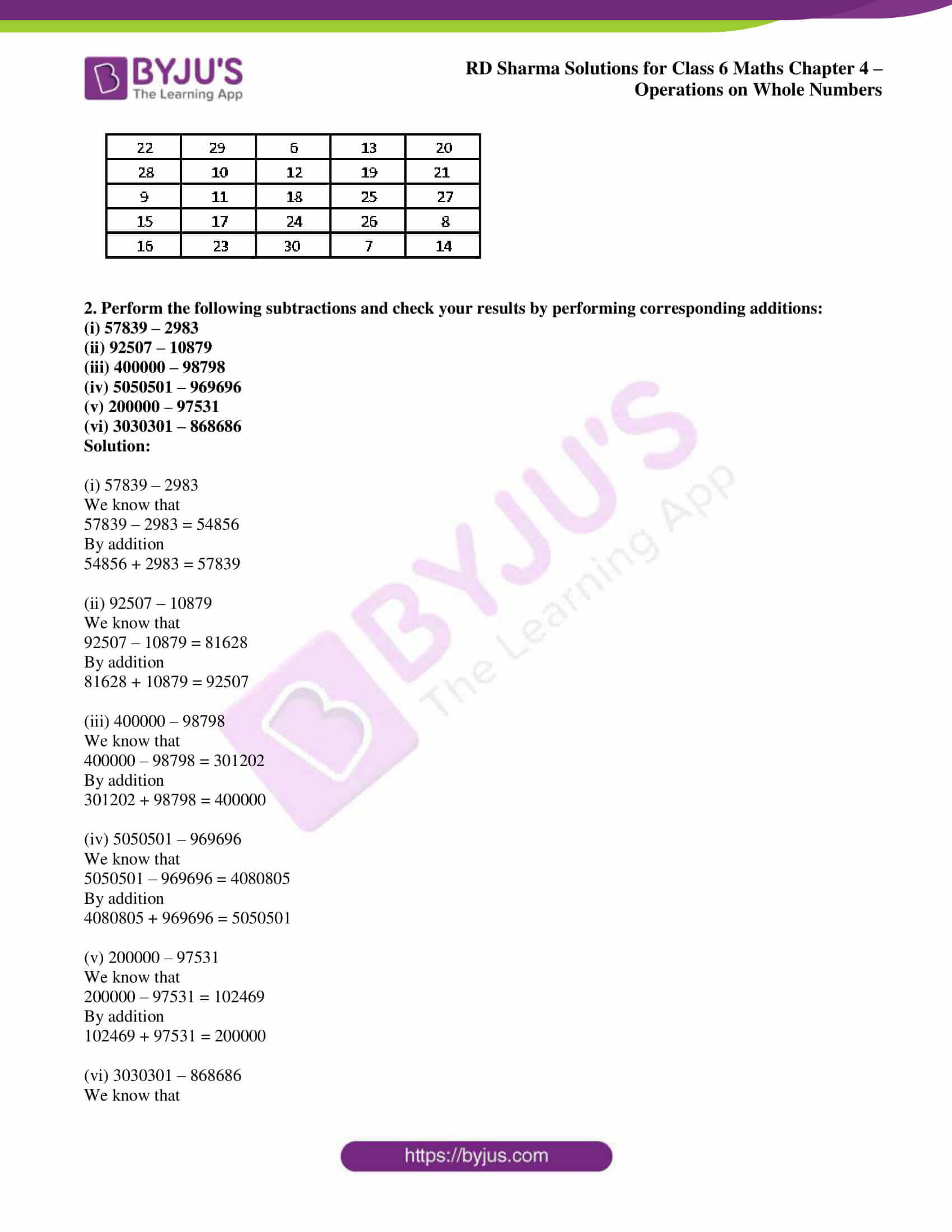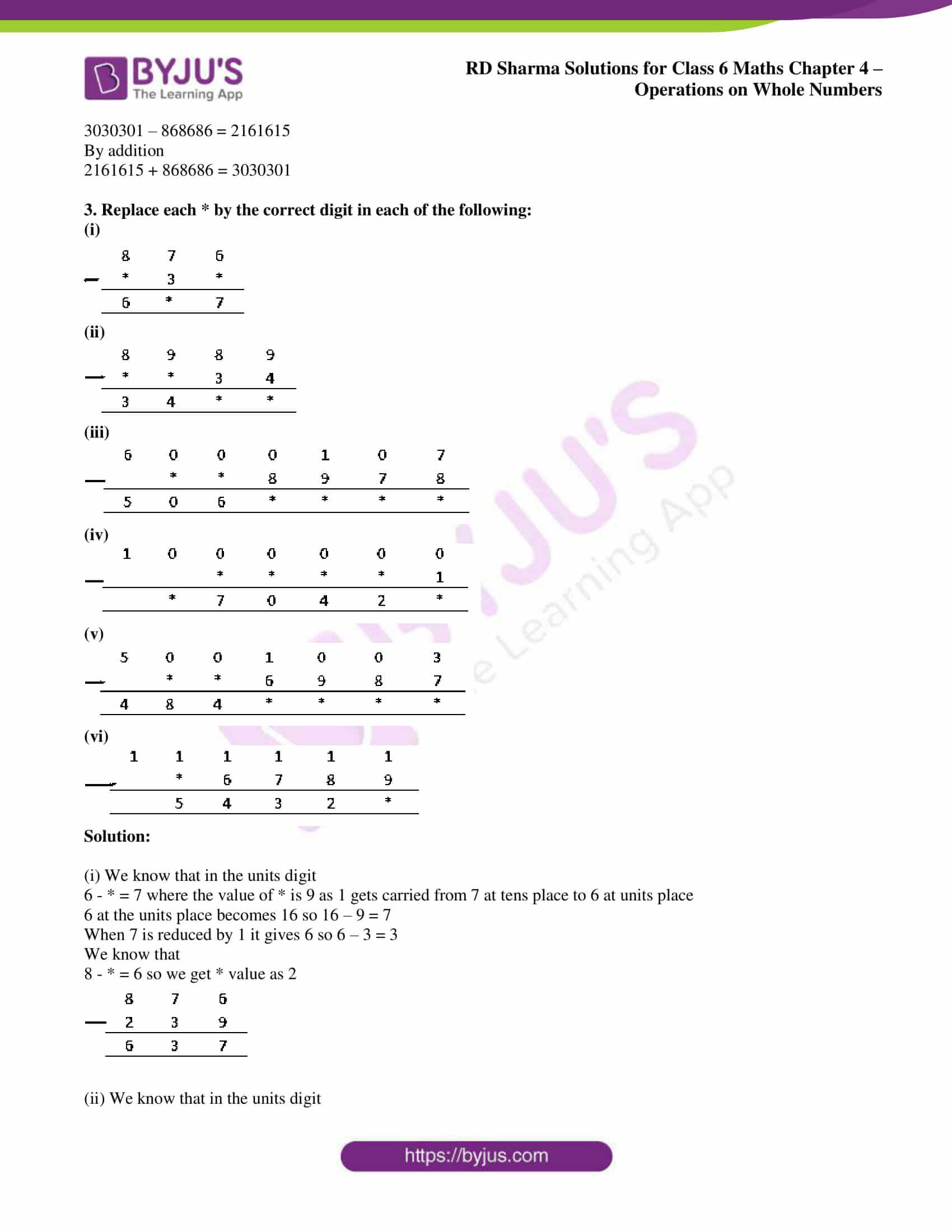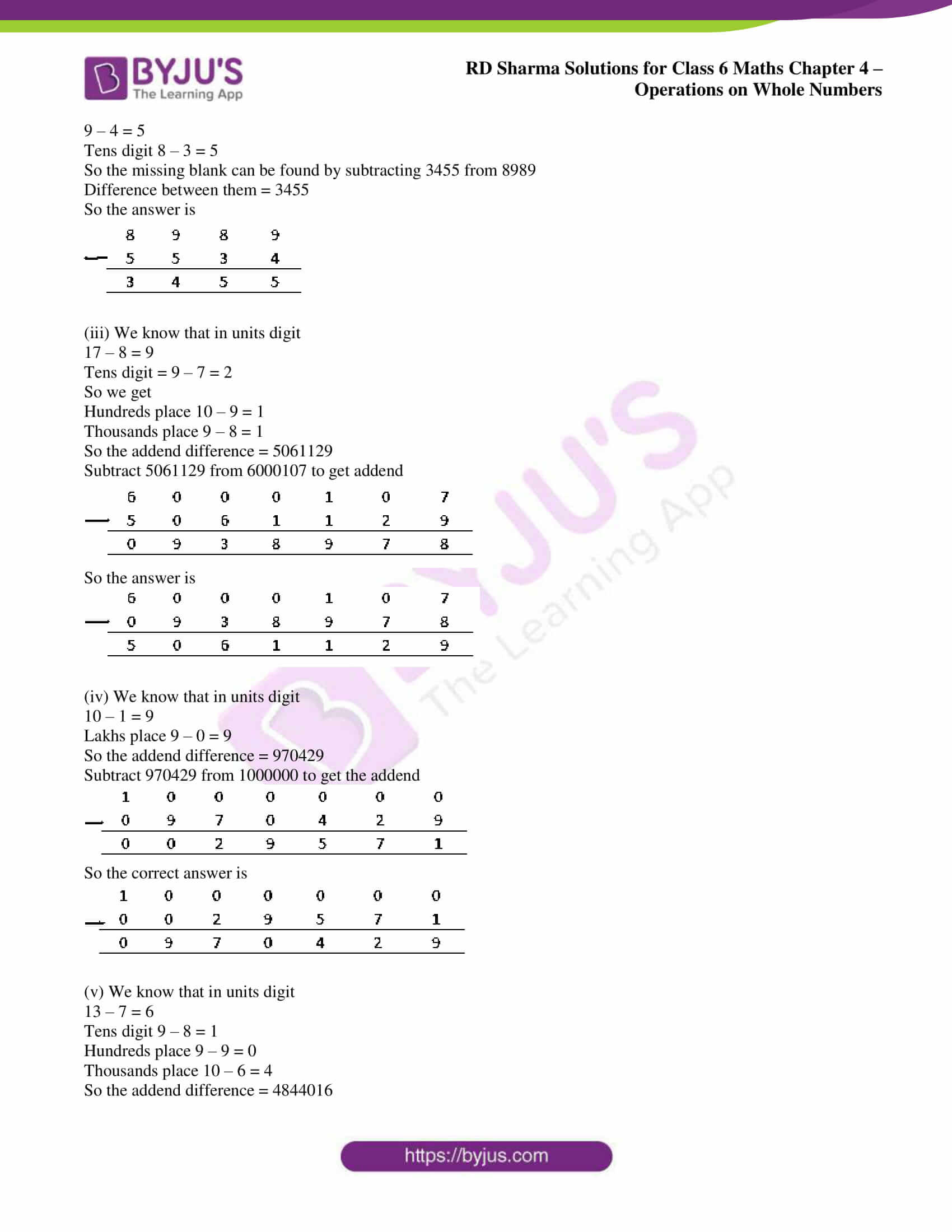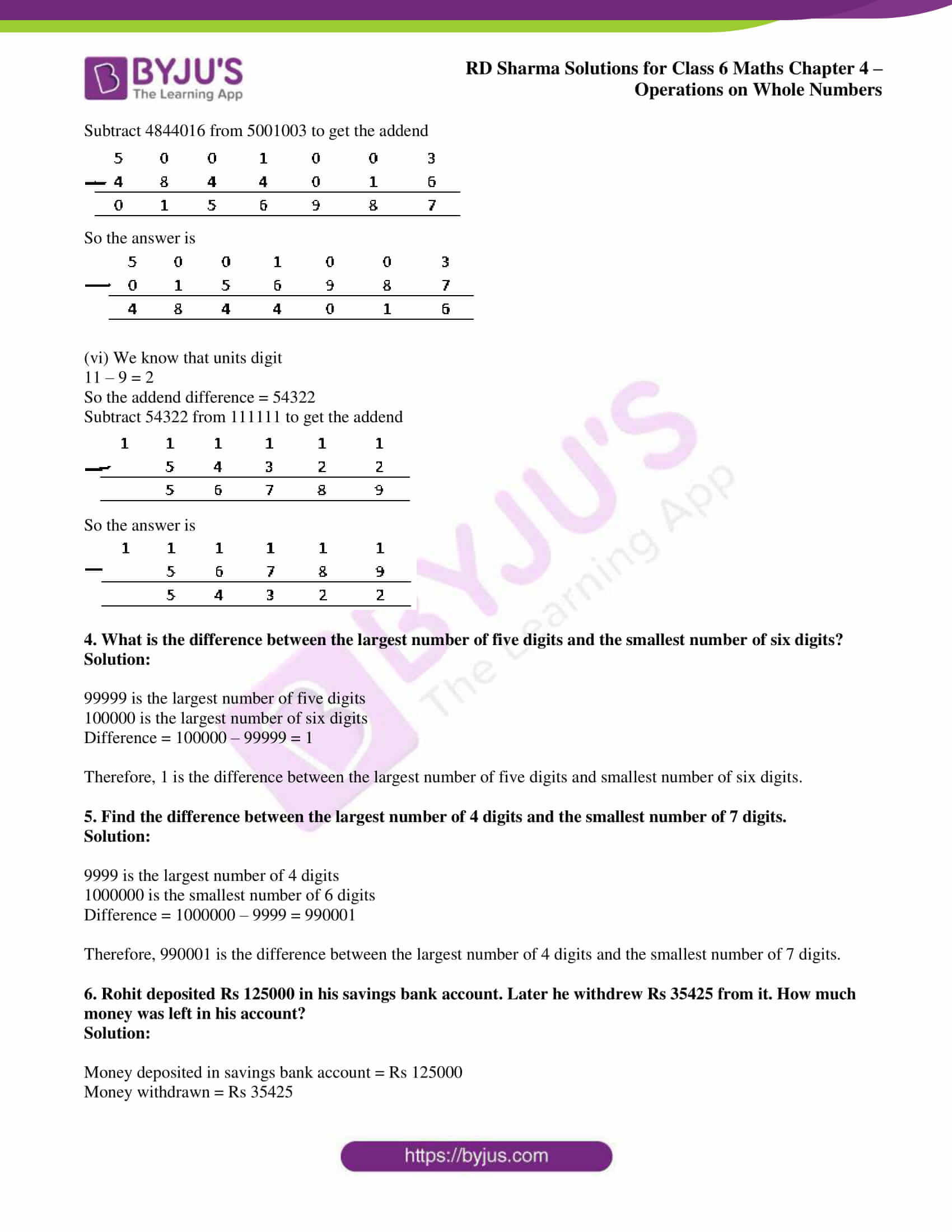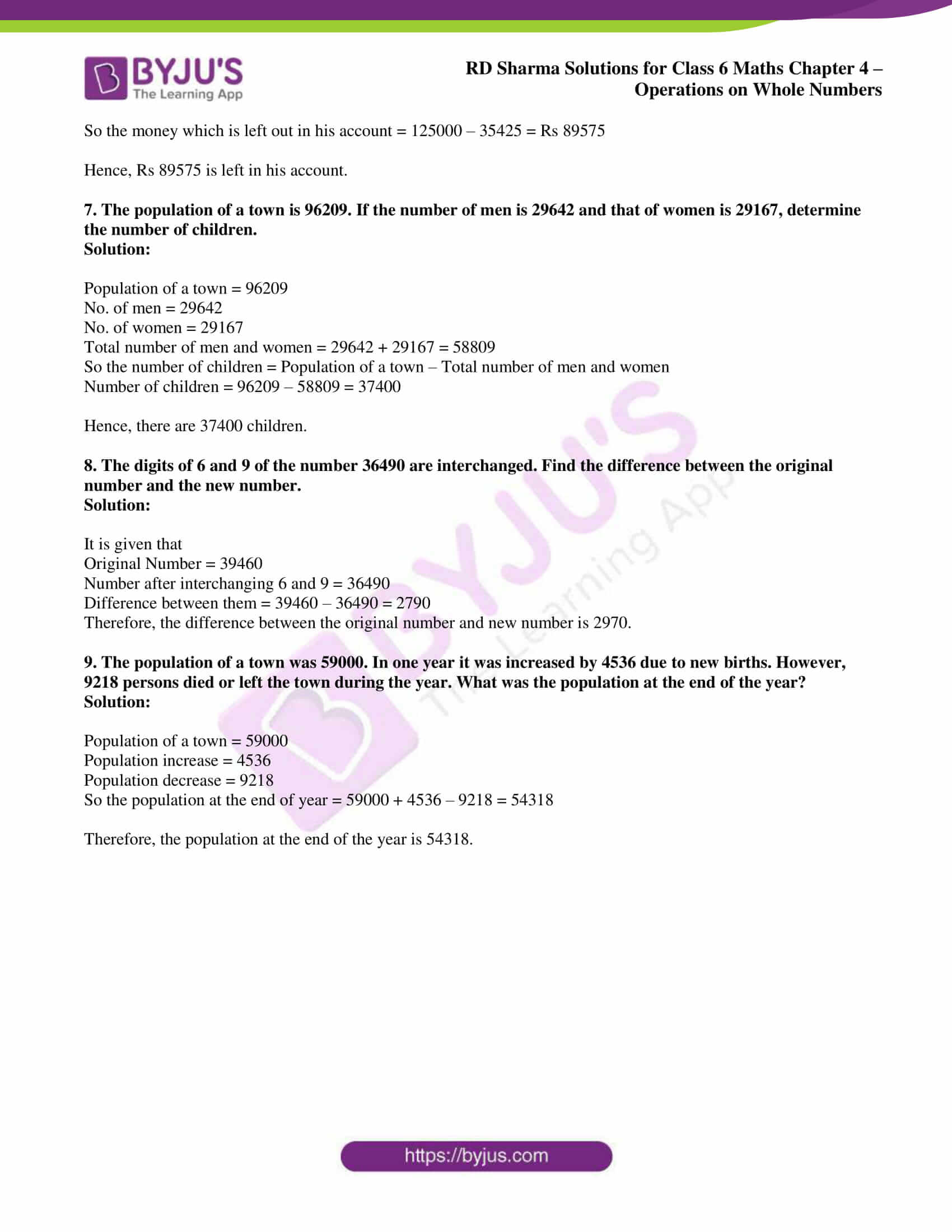## Access answers to Maths RD Sharma Solutions for Class 6 Chapter 4: Operations on Whole Numbers Exercise 4.2

1. A magic square is an array of numbers having the same number of rows and columns and the sum of numbers in each row, column or diagonal being the same. Fill in the blank cells of the following magic squares:

(i)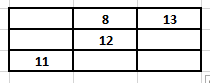(ii)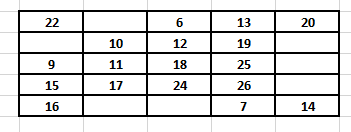Solution:

(i) We know that

Considering diagonal values 13 + 12 + 11 = 36

So we get

No. in the first cell of the first row = 36 – (8 + 13) = 15

No. in the first cell of the second row = 36 – (15 + 11) = 10

No. in the third cell of the second row = 36 – (10 + 12) = 14

No. in the second cell of the third row = 36 – (8 + 12) = 16

No. in the third cell of the third row = 36 – (11 + 16) = 9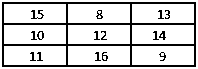(ii) We know that

Considering diagonal values 20 + 19 + 18 + 17 + 16 = 90

So we get

No. in the second cell of the first row = 90 – (22 + 6 + 13 + 20) = 29

No. in the first cell of the second row = 90 – (22 + 9 + 15 + 16) = 28

No. in the fifth cell of the second row = 90 – (28 + 10 + 12 + 19) = 21

No. in the fifth cell of the third row = 90 – (9 + 11 + 18 + 25) = 27

No. in the fifth cell of the fourth row = 90 – (15 + 17 + 24 + 26) = 8

No. in the second cell of the fifth row = 90 – (29 + 10 + 11 + 17) = 23

No. in the third cell of the fifth row = 90 – (6 + 12 + 18 + 24) = 30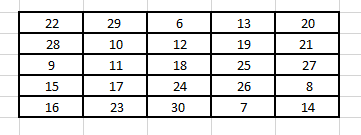2. Perform the following subtractions and check your results by performing corresponding additions:

(i) 57839 – 2983

(ii) 92507 – 10879

(iii) 400000 – 98798

(iv) 5050501 – 969696

(v) 200000 – 97531

(vi) 3030301 – 868686

Solution:

(i) 57839 – 2983

We know that

57839 – 2983 = 54856

54856 + 2983 = 57839

(ii) 92507 – 10879

We know that

92507 – 10879 = 81628

81628 + 10879 = 92507

(iii) 400000 – 98798

We know that

400000 – 98798 = 301202

301202 + 98798 = 400000

(iv) 5050501 – 969696

We know that

5050501 – 969696 = 4080805

4080805 + 969696 = 5050501

(v) 200000 – 97531

We know that

200000 – 97531 = 102469

102469 + 97531 = 200000

(vi) 3030301 – 868686

We know that

3030301 – 868686 = 2161615

2161615 + 868686 = 3030301

3. Replace each * by the correct digit in each of the following:

(i)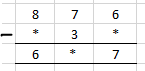(ii)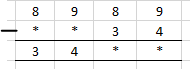(iii)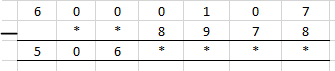(iv)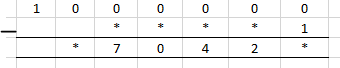(v)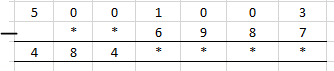(vi)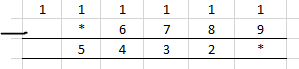Solution:

(i) We know that in the units digit

6 – * = 7 where the value of * is 9 as 1 gets carried from 7 at tens place to 6 at units place

6 at the units place becomes 16 so 16 – 9 = 7

When 7 is reduced by 1 it gives 6 so 6 – 3 = 3

We know that

8 – * = 6 so we get * value as 2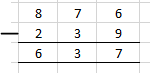(ii) We know that in the units digit

9 – 4 = 5

Tens digit 8 – 3 = 5

So the missing blank can be found by subtracting 3455 from 8989

Difference between them = 3455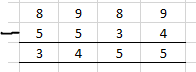(iii) We know that in units digit

17 – 8 = 9

Tens digit = 9 – 7 = 2

So we get

Hundreds place 10 – 9 = 1

Thousands place 9 – 8 = 1

So the addend difference = 5061129

Subtract 5061129 from 6000107 to get addend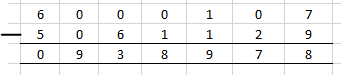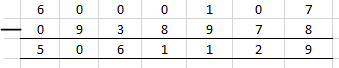(iv) We know that in units digit

10 – 1 = 9

Lakhs place 9 – 0 = 9

So the addend difference = 970429

Subtract 970429 from 1000000 to get the addend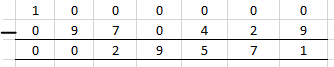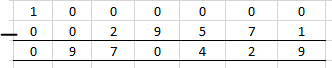(v) We know that in units digit

13 – 7 = 6

Tens digit 9 – 8 = 1

Hundreds place 9 – 9 = 0

Thousands place 10 – 6 = 4

So the addend difference = 4844016

Subtract 4844016 from 5001003 to get the addend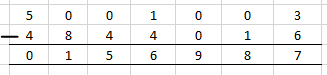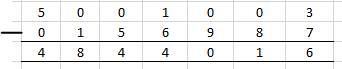(vi) We know that units digit

11 – 9 = 2

So the addend difference = 54322

Subtract 54322 from 111111 to get the addend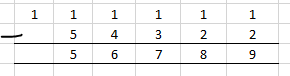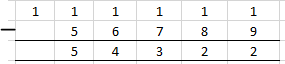4. What is the difference between the largest number of five digits and the smallest number of six digits?

Solution:

99999 is the largest number of five digits

100000 is the largest number of six digits

Difference = 100000 – 99999 = 1

Therefore, 1 is the difference between the largest number of five digits and smallest number of six digits.

5. Find the difference between the largest number of 4 digits and the smallest number of 7 digits.

Solution:

9999 is the largest number of 4 digits

1000000 is the smallest number of 6 digits

Difference = 1000000 – 9999 = 990001

Therefore, 990001 is the difference between the largest number of 4 digits and the smallest number of 7 digits.

6. Rohit deposited Rs 125000 in his savings bank account. Later he withdrew Rs 35425 from it. How much money was left in his account?

Solution:

Money deposited in savings bank account = Rs 125000

Money withdrawn = Rs 35425

So the money which is left out in his account = 125000 – 35425 = Rs 89575

Hence, Rs 89575 is left in his account.

7. The population of a town is 96209. If the number of men is 29642 and that of women is 29167, determine the number of children.

Solution:

Population of a town = 96209

No. of men = 29642

No. of women = 29167

Total number of men and women = 29642 + 29167 = 58809

So the number of children = Population of a town – Total number of men and women

Number of children = 96209 – 58809 = 37400

Hence, there are 37400 children.

8. The digits of 6 and 9 of the number 36490 are interchanged. Find the difference between the original number and the new number.

Solution:

It is given that

Original Number = 39460

Number after interchanging 6 and 9 = 36490

Difference between them = 39460 – 36490 = 2790

Therefore, the difference between the original number and new number is 2970.

9. The population of a town was 59000. In one year it was increased by 4536 due to new births. However, 9218 persons died or left the town during the year. What was the population at the end of the year?

Solution:

Population of a town = 59000

Population increase = 4536

Population decrease = 9218

So the population at the end of year = 59000 + 4536 – 9218 = 54318

Therefore, the population at the end of the year is 54318.

### RD Sharma Solutions for Class 6 Maths Chapter 4 – Operations on Whole Numbers Exercise 4.2

RD Sharma Solutions Class 6 Maths Chapter 4 Operations on Whole Numbers Exercise 4.2 explains properties of subtraction and problem solving methods based on CBSE syllabus.

### Key features of RD Sharma Solutions for Class 6 Maths Chapter 4: Operations on Whole Numbers Exercise 4.2

• The solutions prepared by subject experts improve conceptual knowledge among the students.
• Huge number of problems present before each exercise help students in understanding the methods of solving them.
• The exercise wise problems are made available in PDF format which can be downloaded for reference purpose.
• Self study methods are improved among students which significantly contributes to their success in higher classes.